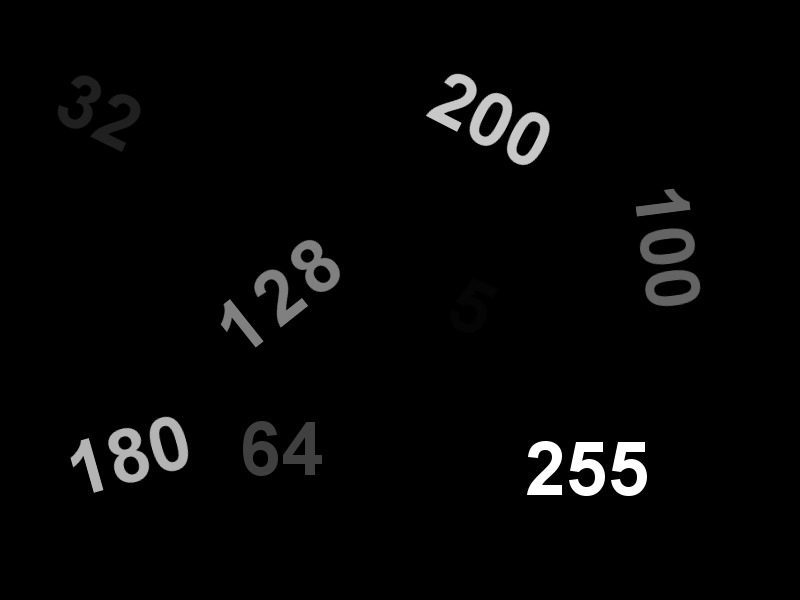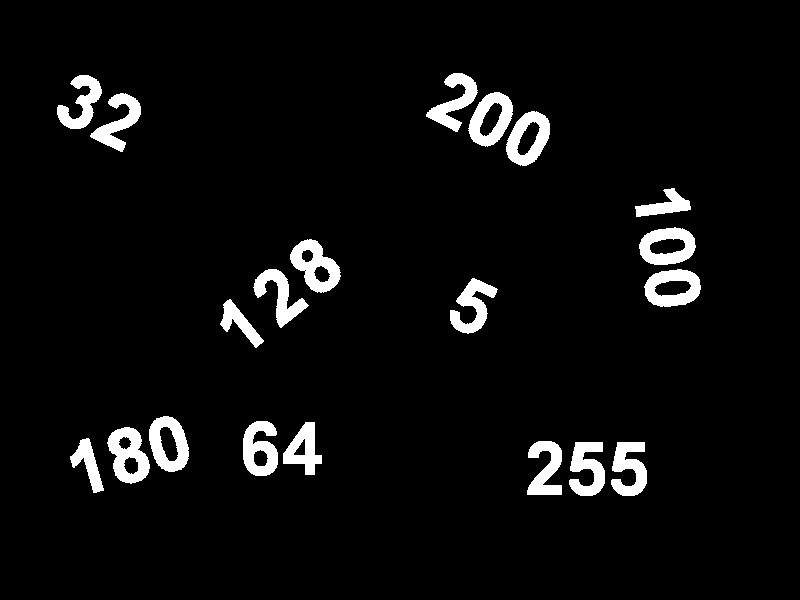OpenCV

# OpenCV Threshold ( Python , C++ )

## What is image thresholding ?

Carefully observe image below (Figure 1). How many numbers do you see ? Most of you will see the numbers : 32 (oh yeah, look carefully), 64, 100,  128, 180, 200 and 255. But there is more to the image than what meets the eye.Figure 1 : Hover over to see thresholded image

Hover over the image to see a thresholded version of the original image. You will notice that all the numbers look completely white ( i.e. they have a grayscale value of 255 ) and there is an extra number 5. You did not notice the number 5 in the original image because its grayscale value was, well 5. In fact all the numbers in the original image have a grayscale value equal to the number. Therefore 255 is the brightest and 5 is the darkest.

Reading numbers in the thresholded image is way easier than reading numbers in the original image. Not surprisingly a text recognition algorithm will find the thresholded image in our example much easier to process than the original image.

In Computer Vision when you make a task easier for humans, you typically make it easier for computer algorithms as well.

All thresholding algorithms take a source image (src) and a threshold value (thresh) as input and produce an output image (dst) by comparing the pixel value at source pixel ( x , y ) to the threshold. If src ( x , y ) > thresh , then dst ( x , y ) is assigned a some value. Otherwise dst ( x , y ) is assigned some other value.

In its simplest form of thresholding is called Binary Thresholding. In addition to the source image (src) and threshold value (thresh), it takes another input parameter called maximum value ( maxValue ). At each pixel location (x,y) it compares the pixel value src ( x , y ) to thresh. If src ( x , y ) is greater than thresh, it sets the value of the destination image pixel dst ( x , y ) to maxValue, otherwise it sets it to zero. Here is what the pseudo code looks like

 1 2 3 4 5 `# Simple threshold function pseudo code` `if` `src(x,y) > thresh` `  ``dst(x,y) ``=` `maxValue` `else` `  ``dst(x,y) ``=` `0`

More generally, there are many types of thresholding based on different threshold rules applied to src ( x , y ) to get dst ( x , y ). Let’s look at the various threshold types available in OpenCV.

## Threshold Examples : C++ and Python

### Input Image

In the following examples we will use this image as input. Click on Figure 2 to download the image in PNG format. The input image contains numbers written with intensity ( grayscale value ) equal to the number itself. E.g. the pixel values of the number 200 is 200, and that of 32 is 32. That is why 32 appears much darker than 200 in the image.In every example we will explain the thresholding rule as pseudo code, provide C++ and python example, and the thresholded output image.

### 1. Binary Thresholding ( type = THRESH_BINARY )

This is the most common and the simplest type of thresholding.

Thresholding rule

 1 2 3 4 5 `# Binary Threshold` `if` `src(x,y) > thresh` `  ``dst(x,y) ``=` `maxValue` `else` `  ``dst(x,y) ``=` `0`

Python

 1 2 3 4 5 6 7 8 9 10 11 12 `# import opencv` `import` `cv2` `# Read image` `src ``=` `cv2.imread(``"threshold.png"``, cv2.IMREAD_GRAYSCALE)` `# Set threshold and maxValue` `thresh ``=` `0` `maxValue ``=` `255` `# Basic threshold example` `th, dst ``=` `cv2.threshold(src, thresh, maxValue, cv2.THRESH_BINARY);`

C++

 1 2 3 4 5 6 7 8 9 10 11 12 `using` `namespace` `cv; ` `// Read image` `Mat src = imread(``"threshold.png"``, IMREAD_GRAYSCALE);` `Mat dst;` `// Set threshold and maxValue` `double` `thresh = 0;` `double` `maxValue = 255; ` `// Binary Threshold` `threshold(src,dst, thresh, maxValue, THRESH_BINARY);`

Result of Binary Threshold

Figure 3 shows the result of applying binary thresholding to the input image withthresh = 0 and maxValue = 255.Changing thresh to 127 removes all numbers less than or equal to 127.Changing maxValue to 128, sets the value of the thresholded regions to 128.### 2. Inverse Binary Thresholding ( type = THRESH_BINARY_INV )

Inverse binary thresholding is just the opposite of binary thresholding. The destination pixel is set to zero if the corresponding source pixel is greater than the threshold, and to maxValue if the source pixel is less than the threshold.

Thresholding rule

 1 2 3 4 5 `# Inverse Binary Threshold` `if` `src(x,y) > thresh` `  ``dst(x,y) ``=` `0` `else` `  ``dst(x,y) ``=` `maxValue`

Python

 `th, dst ``=` `cv2.threshold(src, thresh, maxValue, cv2.THRESH_BINARY_INV)`

C++

 `threshold(src,dst, thresh, maxValue, THRESH_BINARY_INV);`

Result of Inverse Binary Threshold

Notice that the result of inverse binary thresholding shown in Figure 6 is exactly the inverse of Figure 4.### 3. Truncate Thresholding ( type = THRESH_TRUNC )

In this type of thresholding, the destination pixel is set to the threshold ( thresh ) if the source pixel value is greater than the threshold. Otherwise it is set to the source pixel value. maxValue is ignored.

Thresholding rule

 1 2 3 4 5 `# Truncate Threshold` `if` `src(x,y) > thresh` `  ``dst(x,y) ``=` `thresh` `else` `  ``dst(x,y) ``=` `src(x,y)`

Python

 `th, dst ``=` `cv2.threshold(src, thresh, maxValue, cv2.THRESH_TRUNC)`

C++

 `threshold(src,dst, thresh, maxValue, THRESH_TRUNC);`

Result of Truncate Thresholding

Figure 7 shows the result of applying Truncate Thresholding to the input image. Note that all values above the threshold ( 127 ) are set to 127, and all values less than or equal to 127 are unchanged. maxValue is ignored.### 4. Threshold to Zero ( type = THRESH_TOZERO )

In this kind of thresholding, the destination pixel value is set to the corresponding source pixel value if the source pixel value is greater than the threshold. Otherwise it is set to zero. maxValue is ignored.

Thresholding rule

 1 2 3 4 5 `# Threshold to Zero` `if` `src(x,y) > thresh` `  ``dst(x,y) ``=` `src(x,y)` `else` `  ``dst(x,y) ``=` `0`

Python

 1 `th, dst ``=` `cv2.threshold(src, thresh, maxValue, cv2.THRESH_TOZERO)`

C++

 1 `threshold(src,dst, thresh, maxValue, THRESH_TOZERO);`

Result of Threshold to Zero### 5. Inverted Threshold to Zero ( type = THRESH_TOZERO_INV)

In this kind of thresholding, the destination pixel value is set to zero if the source pixel value is greater than the threshold. Otherwise it is set to the source pixel value. maxValue is ignored.

Thresholding rule

 1 2 3 4 5 `# Inverted Threshold to Zero` `if` `src(x,y) > thresh` `  ``dst(x,y) ``=` `0` `else` `  ``dst(x,y) ``=` `src(x,y)`

Python

 1 `th, dst ``=` `cv2.threshold(src, thresh, maxValue, cv2.THRESH_TOZERO_INV)`

C++

 1 `threshold(src,dst, thresh, maxValue, THRESH_TOZERO_INV);`

Result of Inverted Threshold to Zero

Figure 9 shows the result of applying Inverted Threshold to Zero to the input image. The numbers below the threshold retain their grayscale value, and the numbers above the threshold are 0 except for the boundary. The artifacts on the boundary are because of the fact that the pixel values at the boundary transition from 0 to the value of the number over a few pixels. So some of the boundary pixels are below threshold.